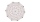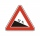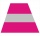# Cuboid face diagonals

The lengths of the cuboid edges are in the ratio 1: 2: 3. Will the lengths of its diagonals be the same ratio?

The cuboid has dimensions of 5 cm, 10 cm, and 15 cm. Calculate the size of the wall diagonals of this cuboid.

Result

y =  0

#### Solution:

$a = 5 \ \\ b = 10 \ \\ c = 15 \ \\ \ \\ a:b:c = 1:2:3 \ \\ \ \\ u_{ 1 } = \sqrt{ a^2+b^2 } = \sqrt{ 5^2+10^2 } = 5 \ \sqrt{ 5 } \doteq 11.1803 \ \\ u_{ 2 } = \sqrt{ a^2+c^2 } = \sqrt{ 5^2+15^2 } = 5 \ \sqrt{ 10 } \doteq 15.8114 \ \\ u_{ 3 } = \sqrt{ b^2+c^2 } = \sqrt{ 10^2+15^2 } = 5 \ \sqrt{ 13 } \doteq 18.0278 \ \\ \ \\ k_{ 1 } = u_{ 1 }:u_{ 2 } = 11.1803:15.8114 \doteq 0.7071 \ \\ k_{ 2 } = u_{ 3 }:u_{ 2 } = 18.0278:15.8114 \doteq 1.1402 \ \\ k_{ 3 } = u_{ 3 }:u_{ 1 } = 18.0278:11.1803 \doteq 1.6125 \ \\ \ \\ k_{ 1 },k_{ 2 },k_{ 3 } \ne 1,2,3 \ \\ \ \\ y = 0$

Leave us a comment of this math problem and its solution (i.e. if it is still somewhat unclear...):Be the first to comment!#### Following knowledge from mathematics are needed to solve this word math problem:

Pythagorean theorem is the base for the right triangle calculator.

## Next similar math problems:

1. Faces diagonalsIf the diagonals of a cuboid are x, y, and z (wall diagonals or three faces) respectively than find the volume of a cuboid. Solve for x=1.2, y=1.7, z=1.45
2. Wall and body diagonalsCalculate the lengths of the wall and body diagonals of the cuboid with edge dimensions of 0.5 m, 1 m, and 2 m
3. Body diagonalCalculate the volume of a cuboid whose body diagonal u is equal to 6.1 cm. Rectangular base has dimensions of 3.2 cm and 2.4 cm
4. Space diagonalThe space diagonal of a cube is 129.91 mm. Find the lateral area, surface area and the volume of the cube.
5. Sides of right angled triangleOne leg is 1 m shorter than the hypotenuse, and the second leg is 2 m shorter than the hypotenuse. Find the lengths of all sides of the right-angled triangle.
6. A rhombusA rhombus has sides of length 10 cm, and the angle between two adjacent sides is 76 degrees. Find the length of the longer diagonal of the rhombus.
7. The trapeziumThe trapezium is formed by cutting the top of the right-angled isosceles triangle. The base of the trapezium is 10 cm and the top is 5 cm. Find the area of trapezium.
8. Isosceles triangle 10In an isosceles triangle, the equal sides are 2/3 of the length of the base. Determine the measure of the base angles.
9. Uphill gardenI have a garden uphill, increasing from 0 to 4.5 m for a length of 25 m, how much is the climb in percent?
10. Medians in right triangleIt is given a right triangle, angle C is 90 degrees. I know it medians t1 = 8 cm and median t2 = 12 cm. .. How to calculate the length of the sides?
11. Two chordsCalculate the length of chord AB and perpendicular chord BC to circle if AB is 4 cm from the center of the circle and BC 8 cm from the center of the circle.
12. Angles of elevationFrom points A and B on level ground, the angles of elevation of the top of a building are 25° and 37° respectively. If |AB| = 57m, calculate, to the nearest meter, the distances of the top of the building from A and B if they are both on the same side of t
13. Coordinates of square verticesI have coordinates of square vertices A / -3; 1/and B/1; 4 /. Find coordinates of vertices C and D, C 'and D'. Thanks Peter.
14. The hemisphereThe hemisphere container is filled with water. What is the radius of the container when 10 liters of water pour from it when tilted 30 degrees?
15. A concrete pedestalA concrete pedestal has a shape of a right circular cone having a height of 2.5 feet. The diameter of the upper and lower bases are 3 feet and 5 feet, respectively. Determine the lateral surface area, total surface area, and the volume of the pedestal.
16. Hole's anglesI am trying to find an angle. The top of the hole is .625” and the bottom of the hole is .532”. The hole depth is .250” what is the angle of the hole (and what is the formula)?
17. Secret treasureScouts have a tent in the shape of a regular quadrilateral pyramid with a side of the base 4 m and a height of 3 m. Determine the radius r (and height h) of the container so that they can hide the largest possible treasure.